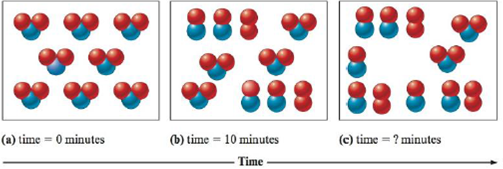# Consider the following representation of the reaction 2NO 2 ( g ) → 2NO( g ) + O 2 ( g ) . Determine the time for the final representation above if the reaction is a. first order b. second order c. zero order### Chemistry: An Atoms First Approach

2nd Edition
Steven S. Zumdahl + 1 other
Publisher: Cengage Learning
ISBN: 9781305079243

#### Solutions

Chapter
Section### Chemistry: An Atoms First Approach

2nd Edition
Steven S. Zumdahl + 1 other
Publisher: Cengage Learning
ISBN: 9781305079243
Chapter 11, Problem 83AE
Textbook Problem
25 views

## Consider the following representation of the reaction 2NO2(g) → 2NO(g) + O2(g).Determine the time for the final representation above if the reaction isa. first orderb. second orderc. zero order

(a)

Interpretation Introduction

Interpretation: The time of completion for the first two representation of decomposition reaction of NO2 is given. The time of final representation for each first, second and zero order is to be calculated.

Concept introduction: The change observed in the concentration of a reactant or a product per unit time is known as the rate of the particular reaction.

To determine: The rate and time of final representation for first order reaction.

To determine: The rate of first order reaction.

### Explanation of Solution

The given reaction is,

2NO2(g)2NO(g)+O2(g)

The diagram (a) represents 0% completion of reaction.

The diagram (b) represents 50% completion of reaction.

The diagram (c) represents 75% completion of reaction.

The half-life is defined as the time required for the concentration of reactant to be reduced to one-half of its initial value. Hence, the half life of the given reaction is 10min .

Formula

The half-life of first order is calculated using the formula,

t12=0.693k

Where,

• t12 is half life

(b)

Interpretation Introduction

Interpretation: The time of completion for the first two representation of decomposition reaction of NO2 is given. The time of final representation for each first, second and zero order is to be calculated.

Concept introduction: The change observed in the concentration of a reactant or a product per unit time is known as the rate of the particular reaction.

To determine: The time of final representation for second order reaction.

(c)

Interpretation Introduction

Interpretation: The time of completion for the first two representation of decomposition reaction of NO2 is given. The time of final representation for each first, second and zero order is to be calculated.

Concept introduction: The change observed in the concentration of a reactant or a product per unit time is known as the rate of the particular reaction.

To determine: The time of final representation for zero order reaction.

### Still sussing out bartleby?

Check out a sample textbook solution.

See a sample solution

#### The Solution to Your Study Problems

Bartleby provides explanations to thousands of textbook problems written by our experts, many with advanced degrees!

Get Started

Find more solutions based on key concepts
Which is true of very low-fat diets? a. They may lack essential fatty acids and certain vitamins. b. They may b...

Nutrition: Concepts and Controversies - Standalone book (MindTap Course List)

Complete the following table:

Chemistry & Chemical Reactivity

Distinguish between scalar and vector quantities.

An Introduction to Physical Science

Chemistry for Today: General, Organic, and Biochemistry

What is genomics?

Human Heredity: Principles and Issues (MindTap Course List)

How does a tendon differ from a ligament?

Human Biology (MindTap Course List)

A nurse measures the temperature of a patient to be 41.5C. (a) What is this temperature on the Fahrenheit scale...

Physics for Scientists and Engineers, Technology Update (No access codes included)

Where would you look for a continental rise? What forms continental rises?

Oceanography: An Invitation To Marine Science, Loose-leaf Versin